## Drilling Fluid Properties

Drilling fluid properties are essential information that everybody should understand.

Density: Mud density is the weight per unit volume of mud and normally it is reported in Pound Per Gallon (PPG). Mud density is used for providing hydrostatic pressure to control well for drilling operation.

Viscosity: It is defined as the internal resistance of fluid flow. There are 2 types of viscosity which are Funnel Viscosity and Plastic Viscosity.

1) Funnel Viscosity: It is time, in seconds for one quart of mud to flow through a Marsh funnel which has a capacity of 946 cm3 (See Figure 1). A quart of water exits the funnel in 26 seconds. This is not a true viscosity, but serves as a qualitative measure of how thick the mud sample is. The funnel viscosity is useful only for relative comparisons.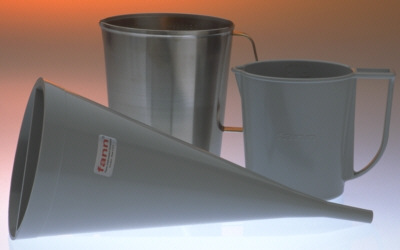Figure 1 Marsh Funnel

2) Plastic Viscosity (PV): A parameter of the Bingham plastic rheological model (See Figure 3). PV is the slope of the shear stress-shear rate plot above the yield point (See Figure 4). Viscometer is equipment to measure Plastic Viscosity (See Figure 2). Plastic Viscosity is derived from the 600 rpm reading minus the 300 rpm reading and PV is in centipoises (cp). A low PV indicates that the mud is capable of drilling rapidly because of the low viscosity of mud exiting at the bit. High PV is caused by a viscous base fluid and by excess colloidal solids. To lower PV, a reduction in solids content can be achieved by dilution.

There are many rheology models shown in Figure 3. Normally Bingham Plastic Model is used to describe mud properties as Plastic Viscosity and Yield Point (See Figure 4).Figure 2 Viscometer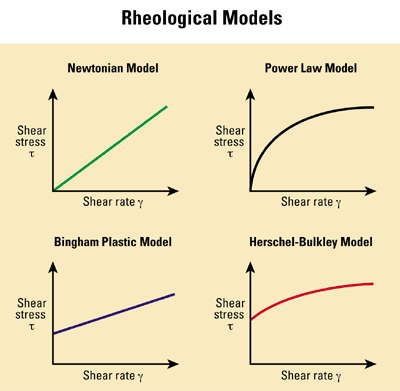Figure 3 Rheology Model (Ref  slb.com)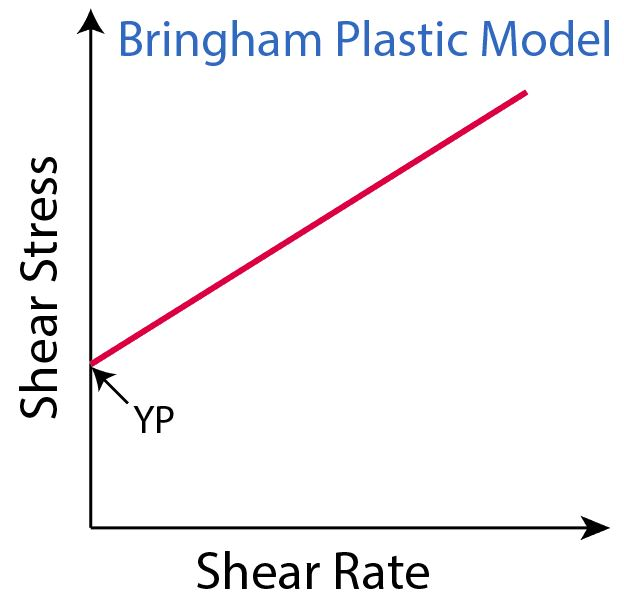Figure 4 Bingham Plastic Model describes PY and VP

Yield Point: Physical meaning is the resistance to initial flow, or the stress required starting fluid movement. The Bingham plastic fluid plots as a straight line on a shear-rate (x-axis) versus shear stress (y-axis) plot, in which YP is the zero-shear-rate intercept (PV is the slope of the line). YP is calculated from 300-rpm and 600-rpm viscometer dial readings by subtracting PV from the 300-rpm dial reading and it is reported as lbf/100 ft2. YP is used to evaluate the ability of mud to lift cuttings out of the annulus. A higher YP implies that drilling fluid has ability to carry cuttings better than a fluid of similar density but lower YP.

Gel Strength: It is the ability of fluid to suspend fluid while mud is in static condition. Before testing gel strength, mud must be agitated for awhile in order to prevent precipitation and then let mud is in static condition for a certain limited time (10 seconds, 10 minutes or maybe 30 minutes) and then open the viscometer at 3 rpm and read the maximum reading value. In a morning report, there are 3 values of gel strength, which are Gel 10sec (lbf/100 ft2), Gel 10 mins (lbf/100 ft2) and Gel 30 mins (lbf/100 ft2).

Ph: This value tells the acid of drilling fluid. Ph paper is used to measure Ph.

Electrical Stability (ES): This value reflects to the stability of emulsion of SDF. If water disperses well in oil phase (good emulsion), the resistivity of drilling fluid will be higher. In contrast, if water disperses badly in oil phase (bad emulsion), the resistivity of drilling fluid will be lower. As the concept above, applied Ohm’s law (V=IR), electricity from the electrical stability meter is emitted in to mud and voltage is measured by the electrical probe. Normally if the measured voltage is higher than 500 volt, the electrical stability is good.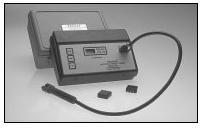Figure 5 Electrical Stability Meter

CaCl2 Concentration: Cl+ can prevent formation swell hence this value must be maintained. It is measured by a titration test by using silver nitrate as titrant with potassium chromate as the endpoint indicator and when titration reaches the equilibrium point mud will change into red.

Retort Test: There are 2 values that are Saraline Water Ratio (SWR) and Solid Content (LGS, Barite) obtained from this testing. Mud is retorted in retort test skid at 950 F for 2 hrs. High temperature can vaporize liquid phase into gas phase and then gas phase will be transferred to a condenser and condense in liquid form. Liquid is stored in a tube that has a level indicator to see how much of water and oil (saraline) extracted. Moreover, solid left in the retort reflects the solid content in mud.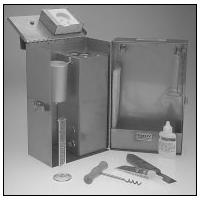Figure 6 Retort Test Skid

HTHP Fluid Loss: This test is conducted for testing fluid loss behavior of mud. Mud is pressed through filter paper located in the HTHP filter press at 300 F with differential pressure at 500 psi for 30 mins. Thickness of filter cake stuck in filter paper should be less than 2 ml.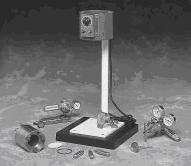Figure 7 HTHP filter press

Reference books:Drilling Fluid Books